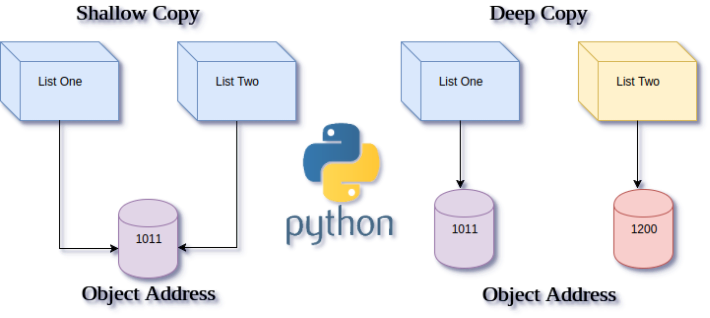• Python常见面试题：Python中的深拷贝和浅拷贝的区别
• 发布于 4天前
• 17 热度
0 评论1.1 使用方法

```list1 = [1, 2, [3, 4]]
list2 = list1.copy()```

1.2 示例

```// 堆代码 duidaima.com
list1 = [1, 2, [3, 4]]
list2 = list1.copy()

print("list1:", list1)
print("list2:", list2)

list1 = 5

print("list1:", list1)
print("list2:", list2)```

```list1: [1, 2, [3, 4]]
list2: [1, 2, [3, 4]]
list1: [1, 2, [5, 4]]
list2: [1, 2, [5, 4]]```

2.1 使用方法

```import copy

list1 = [1, 2, [3, 4]]
list2 = copy.deepcopy(list1)```

2.2 示例

```import copy

list1 = [1, 2, [3, 4]]
list2 = copy.deepcopy(list1)

print("list1:", list1)
print("list2:", list2)

list1 = 5

print("list1:", list1)
print("list2:", list2)```

```list1: [1, 2, [3, 4]]
list2: [1, 2, [3, 4]]
list1: [1, 2, [5, 4]]
list2: [1, 2, [3, 4]]```

• 深拷贝可能会比较耗时，因为它需要递归地复制整个对象树。
• 深拷贝可能会导致循环引用的问题。如果被复制的对象中存在循环引用，那么深拷贝会进入死循环，直到Python的最大递归深度被达到为止。
• 深拷贝可能会导致内存占用过高的问题。如果被复制的对象非常大，那么深拷贝会占用大量的内存。

1. slice操作符
slice操作符可以用于复制列表、元组、字符串等序列类型的对象。例如：
```a = [1, 2, 3, 4]
b = a[:]```

2. list()构造函数
list()构造函数可以用于将其他序列类型的对象转换为列表，并且可以实现浅拷贝。例如：
```a = (1, 2, 3, 4)
b = list(a)```

```a = 123
b = copy.copy(a)
c = copy.deepcopy(a)```

```a = [1, 2, 3]
b = a```